# Polarisation of Light

This is part of the HSC Physics course under the topic Wave Model of Light.

### HSC Physics Syllabus

• conduct investigations quantitatively using the relationship of Malus’ Law I=I_(max) cos^2 θ for plane polarisation of light, to evaluate the significance of polarisation in developing a model for light (ACSPH050, ACSPH076, ACSPH120)

### What is polarisation?

• Electromagnetic waves possess electric and magnetic fields which oscillate perpendicular to each other. However, these fields can be orientated in infinitely many axes – as long as the two fields remain perpendicular. The axis along which the electric field oscillates is referred to as the polarisation axis.
• When a light wave possesses many different polarisation axes, it is unpolarised
• When a light wave possesses only one polarisation axis, it is polarised.

Unpolarised light can be transformed into polarised light

• A polaroid filter is able to polarise light because of its chemical composition. The structure of the filter is arranged in a way such that only light with a particular polarisation axis can pass through.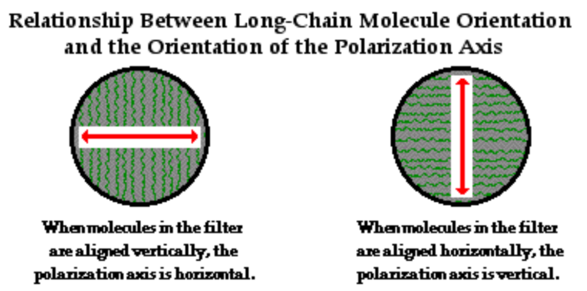The chemical composition of polaroid filters produces a specific transmission axis for light waves. The intensity of light that is allowed to pass through the filter depends on the angle between the filter and wave’s polarisation axis.This can be better understood using the Picket Fence analogy. Light as an electromagnetic wave has oscillating electric and magnetic fields. Polaroid filters exploit the plane of these oscillation by only letting waves whose electric fields are parallel to the transmission axis of the material.

However, the electric fields do not need to be perfectly parallel to pass through, as any component of the electric field that is parallel to the transmission axis will pass. The  perpendicular component will be blocked.

### Malus' Law

Malus’ Law quantitatively characterises this relationship between axis angle and light intensity transmitted or blocked.

In the diagram below, the first polaroid filter has a vertical transmission axis which only permits light with a polarisation axis of the same orientation to pass through.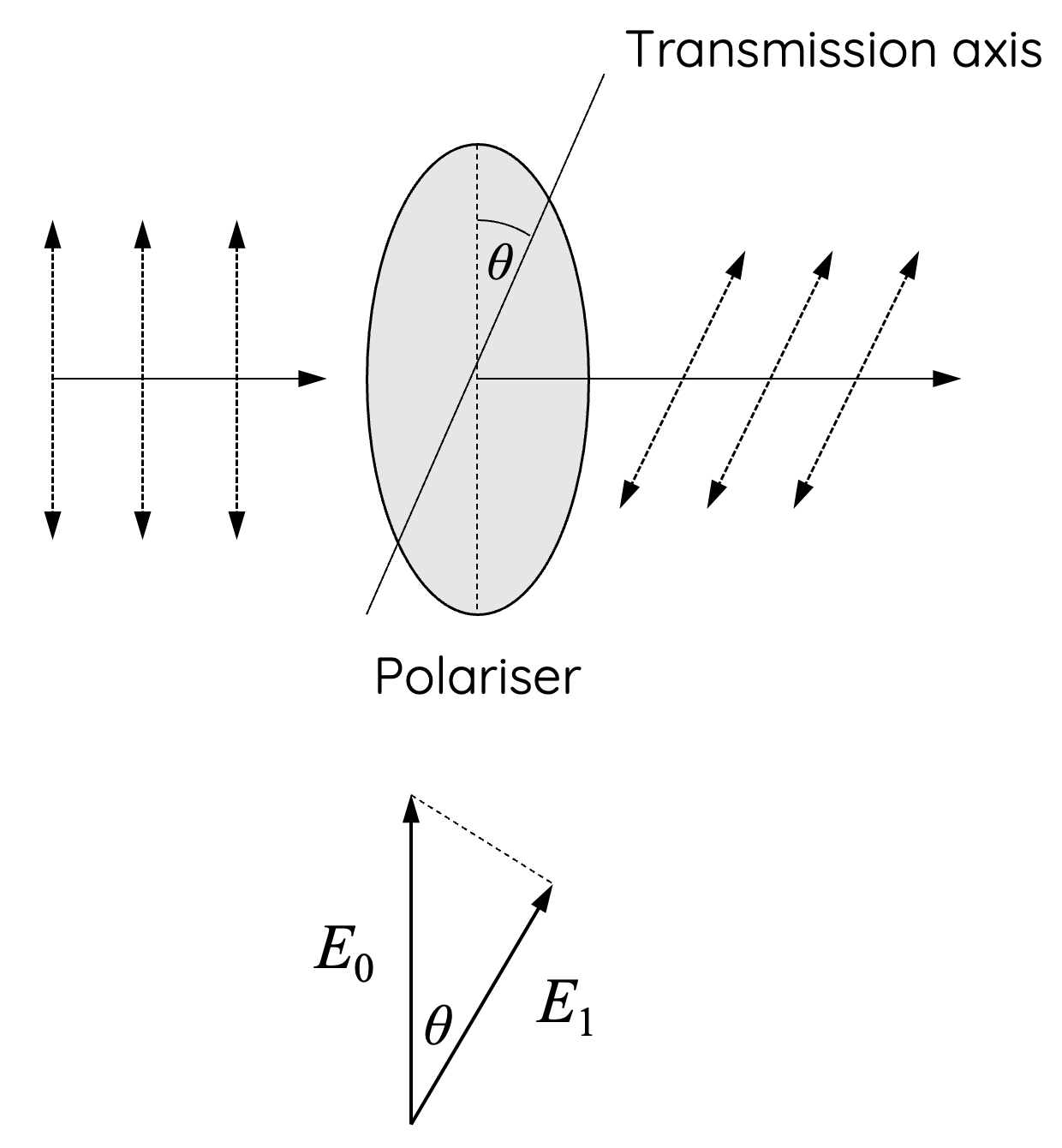Figure 1

Suppose we have a second piece of polaroid whose transmission axis makes an angle θ with that of the first one. The E vector of the light between the polaroids can be resolved into two components, one parallel and one perpendicular to the transmission axis of the second Polaroid (see Figure 1). If we call the direction of transmission of the second polaroid y’,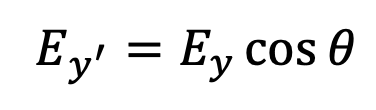Where Ey’ and Ey are the amplitude of the electric field of light passing through polaroid y’ and y respectively.

The intensity of a wave is directly proportional to the square of its amplitude.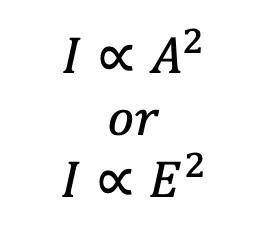Therefore,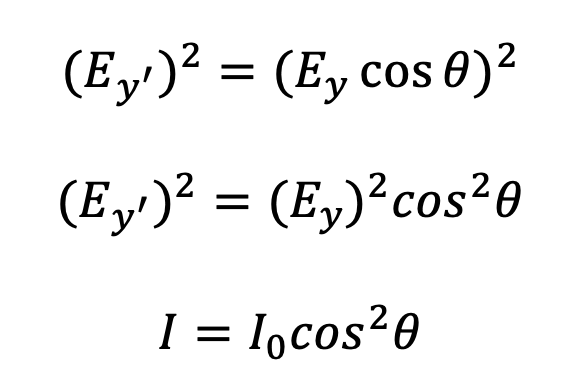Where I and I0 are intensities of light before and after passing through a polariser. θ is the angle between the transmission axis of the polariser and the light’s polarisation axis before entering the polariser.

### Polarisation of Unpolarised Light

Intensity of unpolarised light is reduced by 50%, regardless of the transmission axis of the polariser.This is because the polarisation axes of unpolarised light can be thought as even distributed in all directions (360º).

For angle from 0 to 360º:

$$Average \hspace{0.2cm} value \hspace{0.2cm} of \hspace{0.2cm} cos^2\theta = 0.5$$

### Polarisation supported the transverse wave model of light

Polarising filters have a unique molecular structure that allows only light having a single orientation to pass through.

If a beam of light is allowed to impact a polariser, only light rays oriented parallel to the polarising direction are able to pass through the polariser. If a second polariser is positioned behind the first and oriented in the same direction, then light passing through the first polariser will also pass through the second.However, if the second polariser is rotated at a small angle, the amount of light passing through will be decreased. When the second polariser is rotated so the orientation is perpendicular to that of the first polariser, then none of the light passing through the first polariser will pass through the second.

This effect is easily explained with the electromagnetic wave theory, but no manipulation of the particle theory can explain how light is blocked by the second polariser. Thus, Newton's corpuscular model of light is not adequate in explaining polarisation.

Huygens' wave model of light is unable to explain polarisation as this model describes light as longitudinal waves. Longitudinal waves cannot be polarised.

The effects observed with polarised light were critical to the development of the concept that light consists of transverse waves having components that are perpendicular to the direction of propagation. Each of the transverse components must have a specific orientation direction that enables it to either pass through or to be blocked by a polariser. Only those waves with a transverse component parallel to the polarising filter will pass through, and all others will be blocked.

Previous section: Particle and Wave Model of Light

Next section: Quantum Theory